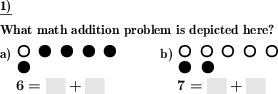Custom math worksheets at your fingertips# Details for problem "Addition up to ten in graphical form"

Quickname: 2763

Elementary School, Primary School.

## Summary

Plus problems up to ten are to be described using marbles.

## Example## Description

An addition task is represented as a series of black and white marbles, the first summand in white, the second one in black. The representation is made in two rows of five balls each. Below this, the problem is shown in the form of a cloze.

The number of tasks can be chosen, as well as the number to be practiced. You can choose between the numbers three to ten and all numbers, a random selection.

Furthermore, it can be determined which parts of the addition task are to be completed.

The first problem can be presented as an example task if desired. This one will contain the answer with the problem.

Download free printable worksheets for this math problem here. The worksheet contains the problems only, the solution sheet includes the answers. Just click on the respective link.

•Worksheet 1Solution sheet with answers
•Worksheet 2Solution sheet with answers
•Worksheet 3Solution sheet with answers

If you can not see the solution sheets for download, they may be filtered out by an ad blocker that you may have installed. If this is the case, please allow ads for this page and reload the page. The solution sheets will then reappear.

• Do these sample worksheets do not really fit?
• Do you need more math worksheets, with a different level of difficulty?
• Would you like to combine different problems on a worksheet and adjust them to your needs?
• As a teacher, you can put together your own worksheets using the automatically generated math problems provided.
With a free initial credit, you can start creating your own math worksheets in a few minutes.

You can try it for free! Register here, to create custom worksheets now!

## Customization options for this problem

Parameter
Possible values
Number of problems
1, 2, 3, 4, 5, 6, 7, 8, 9
Number to practice
All, 3, 4, 5, 6, 7, 8, 9, 10
Fill in...
first summand, second summand, sum, summands, sum,summand 1, sun, summand 2, random one, random two, all
Sample problem
Yes, No

## Similar problems

Remark
Description
As a frames and arrows style calculation chain, successive application the same addition operation
Simple frames and arrows chain task with one elementary arithmetic operation, results are to be filled in blank spaces.